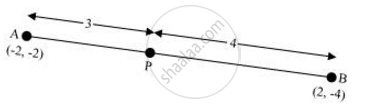# If A and B are (− 2, − 2) and (2, − 4), respectively, find the coordinates of P such that AP = 3/7 AB and P lies on the line segment AB. - Mathematics

If A and B are (− 2, − 2) and (2, − 4), respectively, find the coordinates of P such that AP = 3/7 AB and P lies on the line segment AB.

#### Solution 1The coordinates of point A and B are (−2, −2) and (2, −4) respectively.

Since AP = 3/7 AB

Therefore, AP: PB = 3:4

Point P divides the line segment AB in the ratio 3:4.

Coordinates of P = ((3xx2+4xx(-2))/(3+4), (3xx(-4)+4xx(-2))/(3+4))

= ((6-8)/7, (-12-8)/7)

=(-2/7, -20/7)

#### Solution 2

We have two points A (-2,-2) and B (2,-4). Let P be any point which divides AB as

AP = 3/7 AB

Since,

AB = (AP + BP)

So,

7AP = 3AB

7AP = 3(AP + BP)

4AP = 3BP

(AP)/(BP) = 3/4

Now according to the section formula if any point P divides a line segment joining A(x_1, y_1) and B(x_2, y_2) in the ratio m: n internally than,

P(x,y) = ((nx_1 + mx_2)/(m + n)"," (ny_1 + my_2)/(m + n))

Therefore P divides AB in the ratio 3: 4. So,

P(x,y) = ((3(2) + 4(-2))/(3 + 4)"," (3(-4) + 4(-2))/(3 + 4))

= (-2/7,-20/7)

Concept: Section Formula
Is there an error in this question or solution?
Chapter 7: Coordinate Geometry - Exercise 7.2 [Page 167]

#### APPEARS IN

NCERT Class 10 Maths
Chapter 7 Coordinate Geometry
Exercise 7.2 | Q 8 | Page 167
RD Sharma Class 10 Maths
Chapter 6 Co-Ordinate Geometry
Exercise 6.3 | Q 39 | Page 30

Share